Ch 9. Columns Multimedia Engineering Mechanics BasicColumns FixedColumns EccentricLoads
 Chapter 1. Stress/Strain 2. Torsion 3. Beam Shr/Moment 4. Beam Stresses 5. Beam Deflections 6. Beam-Advanced 7. Stress Analysis 8. Strain Analysis 9. Columns Appendix Basic Math Units Basic Equations Sections Material Properties Structural Shapes Beam Equations Search eBooks Dynamics Fluids Math Mechanics Statics Thermodynamics Author(s): Kurt Gramoll ©Kurt GramollMECHANICS - THEORY

Column Buckling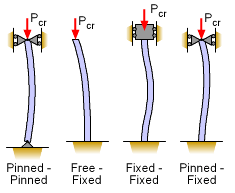Four Basic Column Configurations

Besides the basic pinned-pinned (simply supported) column, there are other types of column boundary conditions. A column can be fixed at one or both ends, and if one end is fixed, then the other can be free.

Each of these basic column types (shown at the left) can be solved in a similar fashion as the pinned-pinned column by developing a differential equation and then solving it using the end conditions. (The derivations are omitted since they are similar to the simply support column case presented previously.)

The basic column types are given below with their critical load equation.

 Pinned - Pinned Free - Fixed Fixed - Fixed Pinned - Fixed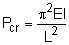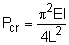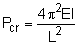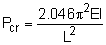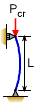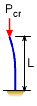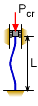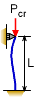Buckling Equations for Critical Load Pcr

 Pinned - Pinned Free - Fixed Fixed - Fixed Pinned - Fixed Le = L Le = 2 L Le= 0.5L Le= 0.7L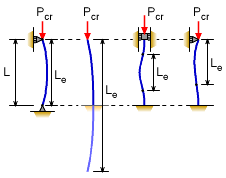Equivalent (or Effective) Lengths
Alternate Buckling Equation Formulation

The four buckling equations given above can be summarized into one equation using the concept of equivalent length (sometimes referred to as effective length). Each of the four buckled column types have a similar buckling shape for a given part of their length. These similar shapes are noted in the diagram as Equivalent Length, Le. Using this equivalent length, Le, a single buckling equation can be used for all four column types.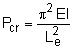Slenderness Ratio, Le/r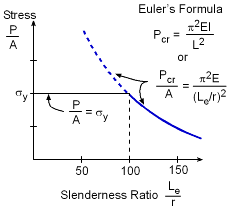For Short Column (Low Slenderness
Ratio) Failure May be Compression

As a column height is reduced, the critical buckling load increases. This relationship can be seen if the basic Euler formula, Pcr = π2EI/L2, is graphed. To make it easier to plot this function, it can be rewritten using the definition of the radius of gyration, r, which is

r = (I/A)0.5    [note, this is not the radius]

Substituting, gives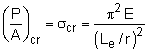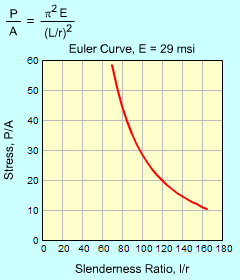Euler Curve for
Various Stiffness Values

This function is only accurate in predicting buckling for slender columns. While each material is different, a slenderness ratio of 100 or greater will generally fail due to buckling. For columns with a slenderness ratio less than 100, the material may fail in compression and the yield stress must be check in addition to buckling.

As demonstrated with the simulation on the left, the value of E, stiffness, has little effect on the general shape of the Euler curve.

Practice Homework and Test problems now available in the 'Eng Mechanics' mobile app
Includes over 400 problems with complete detailed solutions.
Available now at the Google Play Store and Apple App Store.